# Area vs Surface Area Surface Area Surface Area

• Slides: 11
Download presentationArea vs Surface AreaSurface Area • Surface Area is the sum of the area of all of the faces of a 3 -dinemtional figure. • We often use nets to help us determine surface area. • 2 -D (flat) figures have area • 3 -D figures have surface area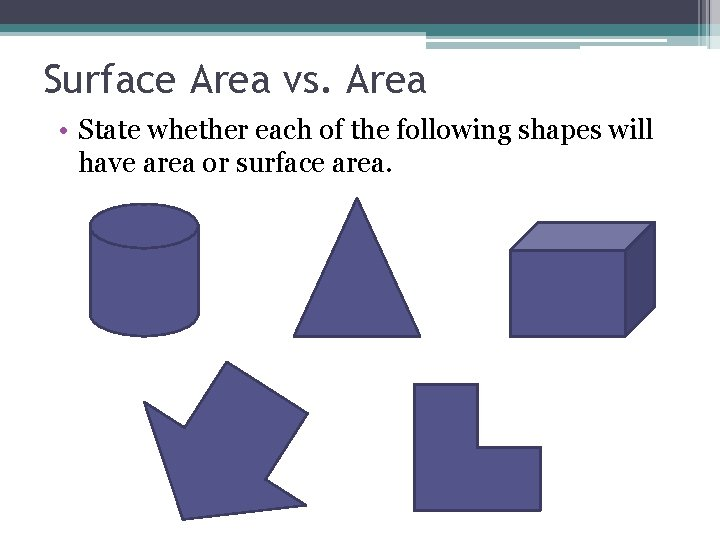Surface Area vs. Area • State whether each of the following shapes will have area or surface area.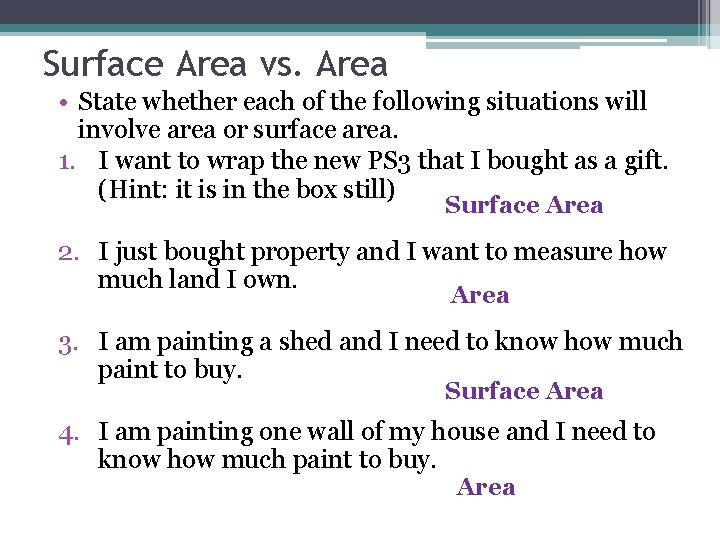Surface Area vs. Area • State whether each of the following situations will involve area or surface area. 1. I want to wrap the new PS 3 that I bought as a gift. (Hint: it is in the box still) Surface Area 2. I just bought property and I want to measure how much land I own. Area 3. I am painting a shed and I need to know how much paint to buy. Surface Area 4. I am painting one wall of my house and I need to know how much paint to buy. AreaFinding Surface Area • We can calculate surface area in two ways: 1. Substitute into a formula. 2. Draw a net and find the area of each section. *If you do not know the formula, the second option will always work!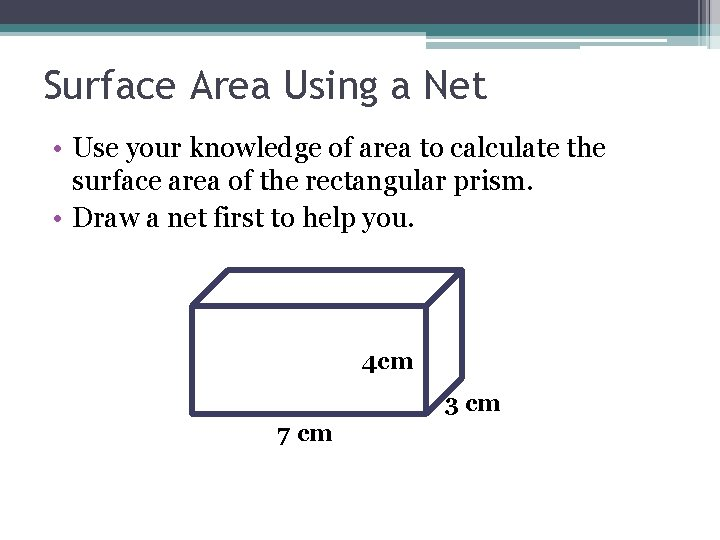Surface Area Using a Net • Use your knowledge of area to calculate the surface area of the rectangular prism. • Draw a net first to help you. 4 cm 3 cm 7 cm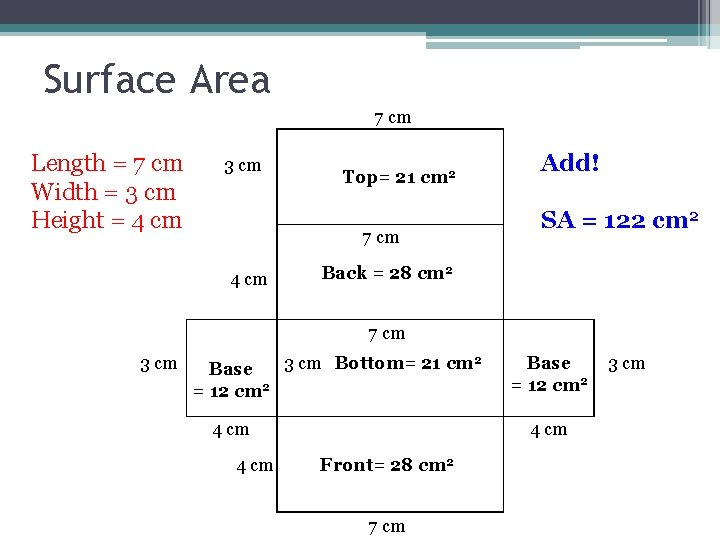Surface Area 7 cm Length = 7 cm Width = 3 cm Height = 4 cm 3 cm Top= 21 cm 2 7 cm 4 cm Add! SA = 122 cm 2 Back = 28 cm 2 7 cm 3 cm Bottom= 21 cm 2 Base = 12 cm 2 4 cm Front= 28 cm 2 7 cm 3 cm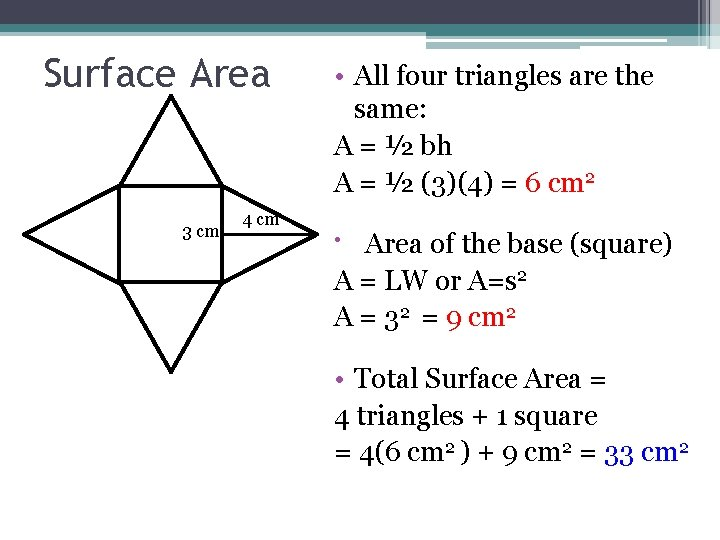Surface Area 3 cm 4 cm • All four triangles are the same: A = ½ bh A = ½ (3)(4) = 6 cm 2 • Area of the base (square) A = LW or A=s 2 A = 32 = 9 cm 2 • Total Surface Area = 4 triangles + 1 square = 4(6 cm 2 ) + 9 cm 2 = 33 cm 2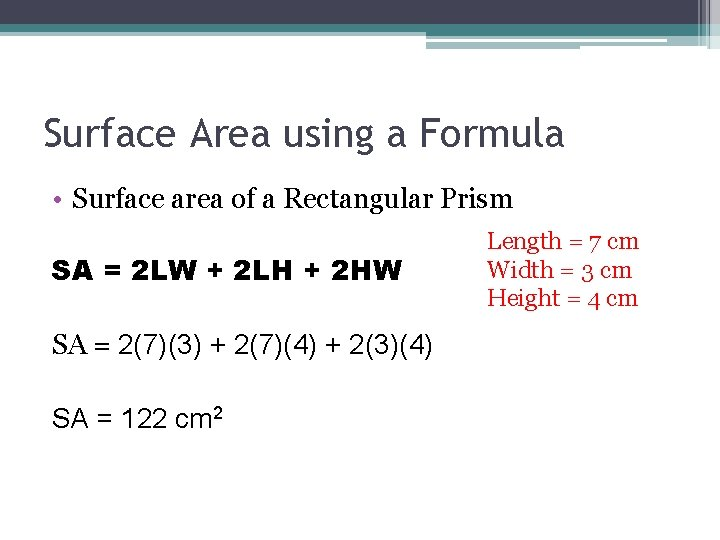Surface Area using a Formula • Surface area of a Rectangular Prism SA = 2 LW + 2 LH + 2 HW SA = 2(7)(3) + 2(7)(4) + 2(3)(4) SA = 122 cm 2 Length = 7 cm Width = 3 cm Height = 4 cm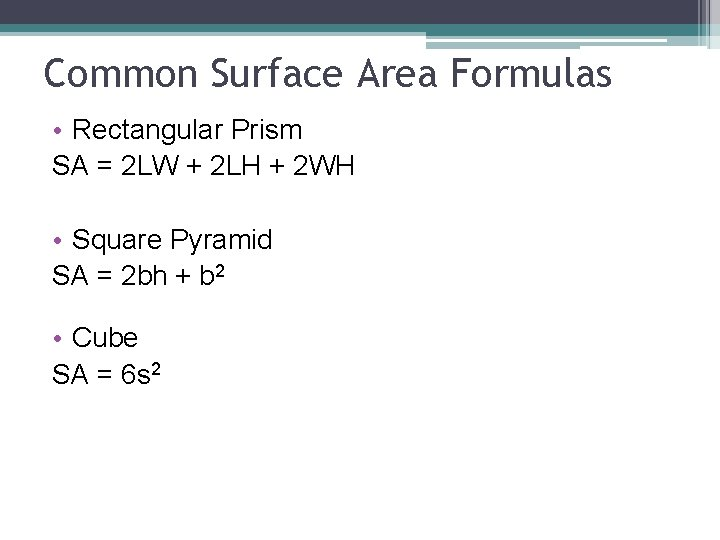Common Surface Area Formulas • Rectangular Prism SA = 2 LW + 2 LH + 2 WH • Square Pyramid SA = 2 bh + b 2 • Cube SA = 6 s 2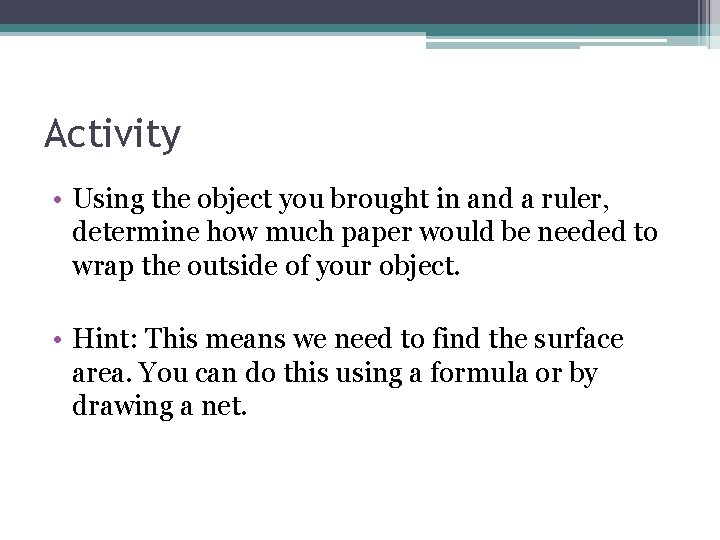Activity • Using the object you brought in and a ruler, determine how much paper would be needed to wrap the outside of your object. • Hint: This means we need to find the surface area. You can do this using a formula or by drawing a net.